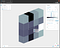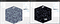# Visual explanation of tensor operations

When you start your machine learning journey, you are very early on hit by new terminology. So let’s see what are tensors and what can we do with them?

If we begin our search on Wikipedia, we’ll encounter something like:

In mathematics, a tensor is an algebraic object that describes a relationship between sets of algebraic objects related to a vector space.

In other places we’ll read that they are synonymous to matrices or tables. Albeit closer, they represent a special case of a 2-dimensional tensor. Similarly, from your maths and physics classes you can remember using vector, which are, similarly, a 1D tensor.

So, from a computer science perspective you can think of them as a storage of N-dimensional data. They can be dense or sparse to indicate whether or not all items within its cells are populated or not. And you can perform standard mathematical operation on between them.

# Defining a tensor

Let’s start by defining a tensor — the numbers up to 16. We can do so in NumPy using just a few lines of code:

We get a terminal output as follows `[0, 1, 2, 3, 4, 5, 6, 7, 8, 9, 10, 11, 12, 13, 14, 15]` . If we want to convert it into a matrix or a 3D tensor we can do so by:

The terminal outputs we receive by printing the values are:

Suddenly this becomes a bit hard to understand, so let’s visualize and inspect the future operations using Efemarai. It works both with numpy and torch tensors and can be used to see any values and rotate around the resulting object.

We can straight away see that these are 2D and 3D tensors and can confirm either by looking at their shape on the top right, or by printing `a_2d.shape` property.

So let’s create another random 2D tensor and perform some operations between them.The random 2D tensor, whose elements are sampled from a standard normal distribution. You can see the distribution of values on the histogram on the top right. Photo by author.

The addition operation between tensors is defined as the element-wise addition. Thus, if they are the same dimensionality, it requires the two tensors to be of the same shape.

# Multiplication

In tensors there are multiple ways to perform a multiplication. It is better described here, but broadly it can be summarized below. Note that the order of the tensors does matter — `a * b` is different than `b * a` .

This is an element-wise product between tensors. In numpy it is abstracted by the symbol `*` .

## Dot

To perform the dot product, we can use the `.dot` operator or the `a @ b` shorthand.

## Cross

The cross product of the vectors X and Y is a vector, perpendicular to both. Let’s say X and Y define a plane. To find the perpendicular to that plane we can calculate

# ReLU operation

In machine learning, it is common to use ReLUs (Rectified Linear Unit) as a non-linearity in processing input data. Neural networks can be abstracted as a sequence of tensor operations interweaved by non-linearities.

The ReLU operator has the mathematical of `y=max(0, x)` . Meaning, it suppresses all negative values in the tensor.

Let’s create a bigger 25x25x25 tensor and apply ReLU to it.Before and after the application of the ReLU operation on the tensor. Photo by Author.

As you can see from the photo above, applying the operation removed all negative values and substituted them by 0s. This can also be see if we look at the distibution of values within the tensor. On the left it follows a standard normal with values ranging between [-3.89, 4.05], whereas on the right there is a large peak around 0 with values [0, 4.05].

# Conclusion

From the examples above we saw that tensors are a useful method for storing information, with different operations that can be applied. Make sure when performing any operations that you inspect the data before and after to confirm your expectations either through printing, plotting moments of the tensor or using a tool like Efemarai. Special note has to be taken in the order of operations and the dimensions of the different tensors.

PhD Machine Learning and Robotics @ University of Edinbrugh

## More from Daniel Angelov

PhD Machine Learning and Robotics @ University of Edinbrugh